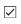# OFDM and Equalization with Prepended Cyclic Prefix

This example prepends a cyclic prefix to OFDM-modulated 16-QAM data. To be effective for equalization the cyclic prefix (CP) length must equal or exceed the channel length.

Define variables for QAM and OFDM processing. Generate symbols, QAM-modulate, OFDM-modulate, and then add a CP to the signal. Multiple OFDM symbols can be processed simultaneously and then serialized.

```bps = 4; % Number of bits per symbol M = 2^bps; % Modulation order nFFT = 128; % Number of FFT bins nCP = 8; % CP length txsymbols = randi([0 M-1],nFFT,1); txgrid = qammod(txsymbols,M,UnitAveragePower=true); txout = ifft(txgrid,nFFT); % To process multiple symbols, vectorize the txout matrix txout = txout(:); txcp = txout(nFFT-nCP+1:nFFT); txout = [txcp; txout];```

Filter the transmission through a channel that adds noise, frequency dependency, and delay to the received signal.

```hchan = [0.4 1 0.4].'; rxin = awgn(txout,40); % Add noise rxin = conv(rxin,hchan); % Add frequency dependency channelDelay = dsp.Delay(1); % Could use fractional delay rxin = channelDelay(rxin); % Add delay```

Add a random offset less than the CP length. An offset setting of zero models perfect synchronization between transmitted and received signals. Any timing offset less than the CP length can be compensated by equalization via an additional linear phase.

```offset = randi(nCP) - 1; % random offset less than length of CP % Remove CP and synchronize the received signal rxsync = rxin(nCP+1+channelDelay.Length-offset:end); rxgrid = fft(rxsync(1:nFFT),nFFT);```

Practical systems require estimation of the channel as part of the signal recovery process. The combination of OFDM and a CP simplifies equalization to a complex scalar for each frequency bin. As long as the latency falls within the length of the CP, synchronization is accomplished by the channel estimator. A control here allows you to experiment by disabling the equalization at receiver front end. Compare the transmitted signal with the receiver output.

```useEqualizer =true; if useEqualizer hfchan = fft(hchan,nFFT); % Linear phase term related to timing offset offsetf = exp(-1i * 2*pi*offset * (0:nFFT-1).'/nFFT); rxgrideq = rxgrid ./ (hfchan .* offsetf); else % Without equalization errors occur rxgrideq = rxgrid; end rxsymbols = qamdemod(rxgrideq,M,UnitAveragePower=true); if max(txsymbols - rxsymbols) < 1e-8 disp("Receiver output matches transmitter input."); else disp("Received symbols do not match transmitted symbols.") end```
```Receiver output matches transmitter input. ```